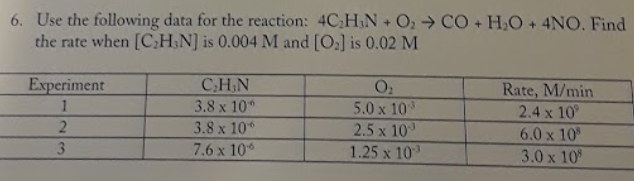# Use the following data for the reaction: 4C2H3N + O2 → CO + H2O + 4NO. Find the rate when [C2H3N] is 0.004 M and [O2] is 0.02 M Experiment 1: [C2H3N] 3.8 x 10^-6 [O2] 5.0 x 10^-3 [Rate, M/min] 2.4 x 10^9 Experiment 2: [C2H3N] 3.8 x 10^-6 [O2] 2.5 x 10^-3 [Rate, M/min] 6.0 x 10^8 Experiment 3: [C2H3N] 7.6 x 10^-6 [O2] 1.25 x 10^-3 [Rate, M/min] 3.0 x 10^8